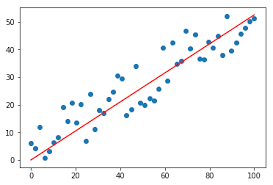1596969720

# Evaluation Metrics for Regression Problems

Hi, today we are going to study about the Evaluation metrics for regression problems. Evaluation Metrics are very important as they tell us, how accurate our model is.

Before we proceed to the evaluation techniques, it is important to gain some intuition.In the above image, we can see that we have plotted a linear curve, but the curve is not perfect as some points are lying above the line & some are lying below the line.

So, how accurate our model is?

The evaluation metrics aim to solve these problems. Now, without wasting time, let’s jump to the evaluation metrics & see the evaluation techniques.

There are 6 evaluation techniques:

1. M.A.E (Mean Absolute Error)

2. M.S.E (Mean Squared Error)

3. R.M.S.E (Root Mean Squared Error)

4. R.M.S.L.E (Root Mean Squared Log Error)

5. R-Squared

Now, let’s discuss these techniques one by one.

### M.A.E (Mean Absolute Error)

It is the simplest & very widely used evaluation technique. It is simply the mean of difference b/w actual & predicted values.

Below, is the mathematical formula of the Mean Absolute Error.

Mean Absolute Error

The Scikit-Learn is a great library, as it has almost all the inbuilt functions that we need in our Data Science journey.

Below is the code to implement Mean Absolute Error

``````from sklearn.metrics import mean_absolute_error

mean_absolute_error(y_true, y_pred)
``````

Here, ‘y_true’ is the true target values & ‘y_pred’ is the predicted target values.

#artificial-intelligence #evaluation-metric #machine-learning #regression #statistics #deep learning

## Buddha Community1596969720

## Evaluation Metrics for Regression Problems

Hi, today we are going to study about the Evaluation metrics for regression problems. Evaluation Metrics are very important as they tell us, how accurate our model is.

Before we proceed to the evaluation techniques, it is important to gain some intuition.In the above image, we can see that we have plotted a linear curve, but the curve is not perfect as some points are lying above the line & some are lying below the line.

So, how accurate our model is?

The evaluation metrics aim to solve these problems. Now, without wasting time, let’s jump to the evaluation metrics & see the evaluation techniques.

There are 6 evaluation techniques:

1. M.A.E (Mean Absolute Error)

2. M.S.E (Mean Squared Error)

3. R.M.S.E (Root Mean Squared Error)

4. R.M.S.L.E (Root Mean Squared Log Error)

5. R-Squared

Now, let’s discuss these techniques one by one.

### M.A.E (Mean Absolute Error)

It is the simplest & very widely used evaluation technique. It is simply the mean of difference b/w actual & predicted values.

Below, is the mathematical formula of the Mean Absolute Error.

Mean Absolute Error

The Scikit-Learn is a great library, as it has almost all the inbuilt functions that we need in our Data Science journey.

Below is the code to implement Mean Absolute Error

``````from sklearn.metrics import mean_absolute_error

mean_absolute_error(y_true, y_pred)
``````

Here, ‘y_true’ is the true target values & ‘y_pred’ is the predicted target values.

#artificial-intelligence #evaluation-metric #machine-learning #regression #statistics #deep learning1598352300

## Regression: Linear Regression

Machine learning algorithms are not your regular algorithms that we may be used to because they are often described by a combination of some complex statistics and mathematics. Since it is very important to understand the background of any algorithm you want to implement, this could pose a challenge to people with a non-mathematical background as the maths can sap your motivation by slowing you down.In this article, we would be discussing linear and logistic regression and some regression techniques assuming we all have heard or even learnt about the Linear model in Mathematics class at high school. Hopefully, at the end of the article, the concept would be clearer.

**Regression Analysis **is a statistical process for estimating the relationships between the dependent variables (say Y) and one or more independent variables or predictors (X). It explains the changes in the dependent variables with respect to changes in select predictors. Some major uses for regression analysis are in determining the strength of predictors, forecasting an effect, and trend forecasting. It finds the significant relationship between variables and the impact of predictors on dependent variables. In regression, we fit a curve/line (regression/best fit line) to the data points, such that the differences between the distances of data points from the curve/line are minimized.

#regression #machine-learning #beginner #logistic-regression #linear-regression #deep learning1592023980

## 5 Regression algorithms: Explanation & Implementation in Python

Take your current understanding and skills on machine learning algorithms to the next level with this article. What is regression analysis in simple words? How is it applied in practice for real-world problems? And what is the possible snippet of codes in Python you can use for implementation regression algorithms for various objectives? Let’s forget about boring learning stuff and talk about science and the way it works.

#linear-regression-python #linear-regression #multivariate-regression #regression #python-programming1605176864

## How to do Problem Solving as a Developer

In this video, I will be talking about problem-solving as a developer.

#problem solving skills #problem solving how to #problem solving strategies #problem solving #developer

1597335660

## Ways to Evaluate Regression Models

The very naive way of evaluating a model is by considering the R-Squared value. Suppose if I get an R-Squared of 95%, is that good enough? Through this blog, Let us try and understand the ways to evaluate your regression model.

### Evaluation metrics;

• Mean/Median of prediction
• Standard Deviation of prediction
• Range of prediction
• Coefficient of Determination (R2)
• Relative Standard Deviation/Coefficient of Variation (RSD)
• Relative Squared Error (RSE)
• Mean Absolute Error (MAE)
• Relative Absolute Error (RAE)
• Mean Squared Error (MSE)
• Root Mean Squared Error on Prediction (RMSE/RMSEP)
• Normalized Root Mean Squared Error (Norm RMSEP)
• Relative Root Mean Squared Error (RRMSEP)

#rmse #machine-learning #regression #data-science #metrics